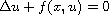Electron. J. Diff. Equ., Vol. 2013 (2013), No. 145, pp. 1-24.

### Existence of multiple solutions to elliptic equations satisfying a global eigenvalue-crossing condition Duong Minh Duc, Loc Hoang Nguyen, Luc Nguyen

Abstract:
We study the multiplicity of solutions to the elliptic equation, under the assumption that f(x,u)/u crosses globally but not pointwise any eigenvalue for every x in a part of the domain, when u varies fromto. Also we relax the conditions on uniform convergence of f(x,s)/s, which are essential in many results on multiplicity for asymptotically linear problems.

Submitted April 10, 2013. Published June 25, 2013.
Math Subject Classifications: 47H11, 55M25, 35J20.
Key Words: Index of critical points; mountain pass type; nonlinear elliptic equations; multiplicity of solutions.

Show me the PDF file (352 KB), TEX file, and other files for this article.Duong Minh Duc University of Sciences - Hochiminh City, Vietnam email: dmduc@hcmuns.edu.vn Loc Hoang Nguyen Department of Mathematics, Ecole Normale Suprieure Paris, France email: lnguyen@dma.ens.fr lu Nguyen Department of Mathematics, Princeton University Princeton, New Jersey 08544, USA email: llnguyen@princeton.edu